Courses
Courses for Kids
Free study material
Free LIVE classes
More## What is Multiplication?

One of the four fundamental mathematical operations, addition, subtraction, multiplication, and division, is multiplication. Multiplication is a process of repeated addition of sets of identically sized objects. Let's use ice cream as an example to better understand.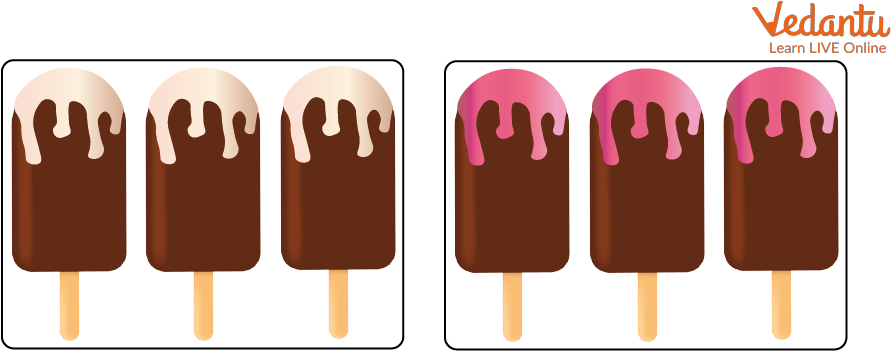Group of Ice-creams

There are two such groupings, each with three ice creams.

6 ice creams total $(3 + 3)$.

You did, however, add two groups of three ice creams. You have therefore multiplied three ice creams by two. It can alternatively be expressed as $(2 \times 3) = 6$.

As we can see, $(2 \times 3)$ and $(3 + 3)$ are equivalent.

Power is a term where multiplication is repeated. The exponent, 2, and base, 5, respectively, are both numbers. The number of times the base is employed as a factor is represented by the exponent.

The technique of repeated addition involves combining equal groups. See the diagram below. There are 3 chickens in each of the 5 groups on the diagram.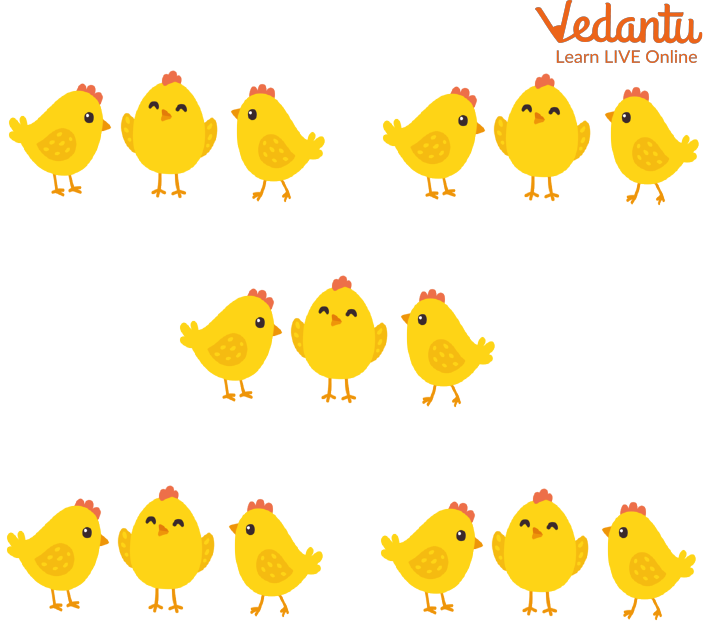Pairs of Chicken

The following repeated addition statement can be used to indicate the overall quantity of oranges: $(3 + 3 + 3 + 3 + 3) \text { or } 15$

Let’s give a look at other repeated addition examples.

Example 1. Calculate the total number of boxes in the figure.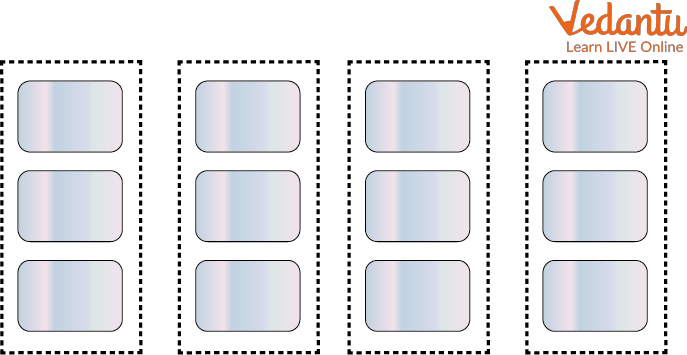Four Group of Three Boxes

Ans: There are 3 boxes in each column and there are 4 columns.

$\text { So, }(3 + 3 + 3 + 3) = (4 \times 3) =12$

Example 2. Each of Raj’s three boxes of markers contains five markers. How many markers does raj have now?

Ans: Each box with five markers indicates that the number five has been repeated three times.

$(5 + 5 + 5) =15 \text { or, }$

This can be represented as an additional formula: $3 \times 5$ equals 15, which is another way to write this as a multiplication phase.

Raj has a total of 15 markers.

Multiplication is a repeated Addition that involves joining like groups together. If the same precise number is repeated, repeated addition can be expressed as multiplication. For example,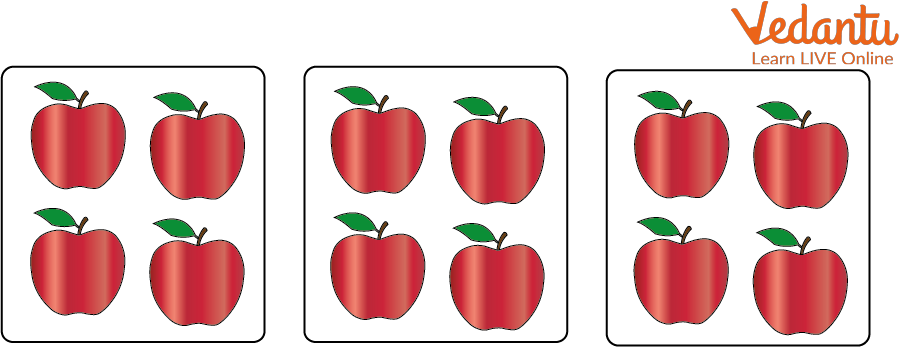Three Group of Four Apples

In the above diagram, three groups of four apples are there. We can also write them as $(4 + 4 + 4) = (3 \times 4) = 12$.

Learn the multiplication facts by repeating the addition. If you are unfamiliar with the facts for $7 \times 3$, for instance, you might find it simpler to calculate $(7 \times 3)$ by writing $(3 + 3 + 3 + 3 + 3 + 3)$ or $(7 + 7 + 7)$; and then gradually adding. It might be useful when dealing with bigger numbers, such as $(5 \times 40)$. Writing $(40 + 40 + 40 + 40 + 40 + 40)$ and then adding the tens is more convenient.

Example: Three muffin packages were bought by Andrew. Four muffins were included in each packet. What number of muffins did Andrew buy?

Ans: In numerical notation, three packages of four muffins each can be written as $(3 \times 4)$ or $(4 + 4 + 4)$, which is 12.

In total, Andrew purchased 12 muffins.

## Practice Question

1. Calculate the total number of boxes in the figure.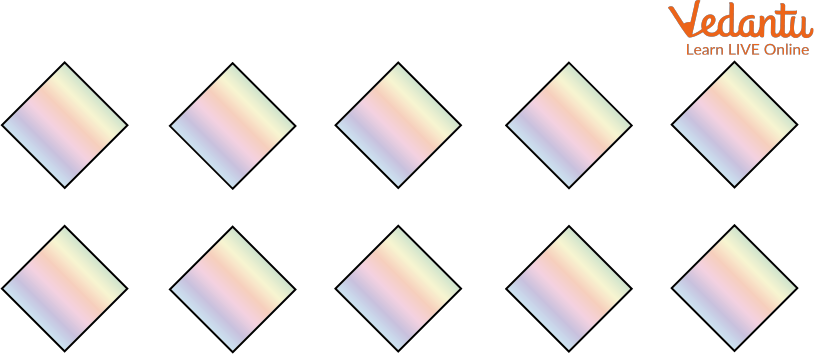Group of Boxes

Ans: 10

2. Two pen pockets were bought by Adda. Four pens were included in each packet. What number of pens did Adda buy?

Ans: 8

## Summary

Multiplication is a process of repeated addition of sets of identically sized objects. The technique of repeated addition involves combining equal groups. Multiplication is repeated addition which involves joining like groups together. When the same precise number is repeated, repeated addition can be expressed as multiplication.

Last updated date: 26th Sep 2023
Total views: 124.2k
Views today: 3.24k

## FAQs on Multiplication is Repeated Addition

1. What is the multiplication formula and what are its guidelines?

Multiplicand x Multiplier = Product is how the multiplication formula is written. The first number is multiplicand (factor). Multiplier: The following figure (factor). The outcome of multiplying the multiplicand and multiplier is the product.

Following are the guidelines:

• Any number multiplied by zero always equals zero.

• Any integer multiplied by one is always the same.

• When multiplying by 10, add a 0 to the original value.

• The product is unaffected by the order of the elements.

• If two numbers with the same signs are multiplied, the products are always positive.

2. What does short multiplication mean and why is multiplication crucial in practical terms?

Columns are used to lay out and calculate multiplication in the short multiplication method. The use of short multiplication, which is an extension of times tables, makes it possible to solve problems more quickly than by repeatedly adding. It is crucial in many ordinary circumstances as well. It might be useful for kids in calculations like allocating money among siblings. There are many circumstances in daily life, including cooking, gardening, and gathering information on the number of people who could benefit from knowing their multiplication tables.